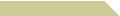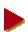HOME COURSES NOTES EXAMS RÉSUMÉ

### DIGIT SUM AND PRIME NUMBERS

The digit sum of a number is the sum of its digits as written in the decimal counting system. For example, the number 19392 has digit sum 24, which we get by adding 1+9+3+9+2=24. We introduce here two classes of numbers which enjoy interesting properties involving their digit sum and a relation with prime numbers.

##Smith Numbers

The number 9405853956, of digit sum 54, factors into prime numbers as

9405853956 = 2 * 2 * 3 * 3 * 23 * 743 * 15289

It just happens that these prime factors together contribute to the same digit sum of 54:

2 + 2 + 3 + 3 + (2 + 3) + (7 + 4 + 3) + (1 + 5 + 2 + 8 + 9) = 54

This digital feature is what makes 9405853956 a Smith number. Of course, this way all prime numbers are Smith numbers in a trivial way, so we agree to restrict the definition of Smith numbers only for composites.

Smith numbers were named in 1982 by Albert Wilansky after his brother in law, whose telephone number has this peculiar property. For further information on Smith numbers, you can go to page A006753 of the Online Encyplopedia of Integer Sequences (OEIS).

Testing an integer N to see if it is a Smith number is quite straightforward once we have the prime factors of N. See our trial division applet to learn one simple algorithm for factoring medium-sized numbers. Our Smith number generator below works for all JavaScript integers, although you would see a delayed response with larger numbers.

##Moran Numbers

Moran numbers appear on page A001101 at OEIS. An example is the number 17727888, which has digit sum 48, and which happens to be one of its own factors, i.e., we get a factorization

17727888 = 48 * 369331

A number with this property is called a Niven number, after the mathematician Ivan M. Niven (1915–1999). What makes 17727888 a Moran number though, is the additional fact that the other factor of 369331 is a prime number!

So to recognize a Moran number N, first we make sure that N is divisible by its digit sum S, and then we test for primality on the quotient N/S. This second task is ideally suited to our former primality testing program.

Here is a JavaScript tool that generates Moran numbers. Feel free to save or modify our source code for your own use.

##But Why Decimal?

We admit that Smith and Moran numbers center upon special properties that are specific to the number system being used, in this case, base 10. So if one of our Smith numbers is expressed in binary, then we cannot ask that the new digit sum would preserve its Smith quality.

On the other hand, a prime number is prime no matter which number base we choose, because primality stands on divisibility properties, not on radix representation. Thus in general, a research work on prime numbers with certain digital restrictions can be hard simply because, presumably, there is no expected correlation between primes and digital patterns.

Understandably, many mathematicians also consider digital problems rather unimportant, since they do not really address the arithmetic structure of the integers. With that, such study is often classified under the topics in Recreational Mathematics.